Solid State & Surface Chemistry

# Solid State & Surface Chemistry

Test Description

## 30 Questions MCQ Test Chemistry Mock Test Series for JEE | Solid State & Surface Chemistry

Solid State & Surface Chemistry for Class 12 2023 is part of Chemistry Mock Test Series for JEE preparation. The Solid State & Surface Chemistry questions and answers have been prepared according to the Class 12 exam syllabus.The Solid State & Surface Chemistry MCQs are made for Class 12 2023 Exam. Find important definitions, questions, notes, meanings, examples, exercises, MCQs and online tests for Solid State & Surface Chemistry below.
Solutions of Solid State & Surface Chemistry questions in English are available as part of our Chemistry Mock Test Series for JEE for Class 12 & Solid State & Surface Chemistry solutions in Hindi for Chemistry Mock Test Series for JEE course. Download more important topics, notes, lectures and mock test series for Class 12 Exam by signing up for free. Attempt Solid State & Surface Chemistry | 30 questions in 45 minutes | Mock test for Class 12 preparation | Free important questions MCQ to study Chemistry Mock Test Series for JEE for Class 12 Exam | Download free PDF with solutions
 1 Crore+ students have signed up on EduRev. Have you?
Solid State & Surface Chemistry - Question 1

### The potential difference between the fixed charged layer and the diffused layer having opposite charge is called:

Solid State & Surface Chemistry - Question 2

### Which one of the following methods is commonly used for destruction of colloid?

Detailed Solution for Solid State & Surface Chemistry - Question 2

Addition of electrolyte brings in coagulation of sol.

Solid State & Surface Chemistry - Question 3

### Select the correct statements : (i) greater is the valency of effective ion in electrolyte more will be coagulation power of electrolyte for a given sol. (ii) greater is the valency of effective ion in electrolyte lesser will be its coagulation value for a given sol. (iii) peptisation is a characteristic property of colloidal solution. (iv) micelle formation occurs only above Kraft’s temperature as well as above critical micelle concentration. (v) lower is the gold number more is protecting power of colloid.

Detailed Solution for Solid State & Surface Chemistry - Question 3

Peptisation is a method to prepare sol.

Solid State & Surface Chemistry - Question 4

How many ‘nearest’ and ‘next nearest’ neighbours respectively potassium have in bcc lattice?

Detailed Solution for Solid State & Surface Chemistry - Question 4

Nearest neighbours are corners & next nearest are body centres of adjacent unit cell, in bcc lattice.

Solid State & Surface Chemistry - Question 5

A binary solid (A+ B-) has a zinc blends structure with B- ions constiuting the lattice and A+ ions occupying 25% tetrahedral holes. The formula of solids is:

Detailed Solution for Solid State & Surface Chemistry - Question 5

No. of B- ions in unit cell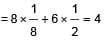Now A+ ions occupies 25% of tetrahedral holes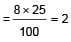Thus, ratio of B- to  AA+ is 2 : 1 or formula is AB2

Solid State & Surface Chemistry - Question 6

Which of the following statement is not correct?

Detailed Solution for Solid State & Surface Chemistry - Question 6

No. of carbon atoms in unit cell of diamond is 8, as it follow ZnS type

Solid State & Surface Chemistry - Question 7

If NaCI is doped with 10-4 mole % of SrCI2, the concentration of cation vacancies will be :

Detailed Solution for Solid State & Surface Chemistry - Question 7

Dropping of SrCI2 to NaCI brings in replacement of two Na+ by each Sr2+ ion, but Sr2+ occupies one lattice point.
This produces one cation vacancy.
No. of cation vacancies = 10-4 mol%
100 mole of NaCI will have cationic vacancy = 10-4
∴ 1 mole of NaCI will have cationic vacancy = 10-4
∴ 100 = 10-6
∴ No. of cationic vacancies
= 10-6 x 6.02 x 1023 = 6.02 x 1017

Solid State & Surface Chemistry - Question 8

AB crystallises in a bcc lattice with edge length ‘a’ equal to 387 pm. The distance between two oppositely charged ions in the lattice is:

Detailed Solution for Solid State & Surface Chemistry - Question 8

For a bcc lattice,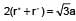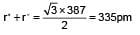Solid State & Surface Chemistry - Question 9

How many unit cells are present in a cube shaped ideal crystal of NaCI of mass 1.00 g?

[Atomic masses Na = 23, CI = 35.5]

Detailed Solution for Solid State & Surface Chemistry - Question 9

Mass of one unit cell = V x d (V is volume, d is density)
= a3 x d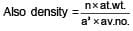∴ Mass of one unit cell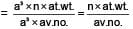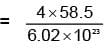(n = 4 for cubic shape)

= 38.87 x 10-23g

∴  No. of unit cell is 1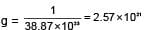Solid State & Surface Chemistry - Question 10

In a compound, atoms of element Y from ccp lattice and those of element X occupy 2/3rd of tetrahedral voids. The formula of  the compound will be:

Detailed Solution for Solid State & Surface Chemistry - Question 10

In a ccp array number of tetrahedral voids is twice the number of atoms.

Solid State & Surface Chemistry - Question 11

Copper crystallises in fcc with a unit cell length of 361 pm. What is the radius of copper atom?

Detailed Solution for Solid State & Surface Chemistry - Question 11

For fcc n = 4

and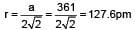Solid State & Surface Chemistry - Question 12

A metallic element crystallises into lattice containing a sequence of layers of ABABABAB......Any packing of spheres leaves out avpoid in the lattice. The empty space in percentage by volume on thid lattice is:

Detailed Solution for Solid State & Surface Chemistry - Question 12

ABABAB...packing has empty space of 48% in sc, 32% in bcc, 26% in hcp and ccp.

Solid State & Surface Chemistry - Question 13

Which statement is wrong?

Detailed Solution for Solid State & Surface Chemistry - Question 13

Hydrogenation of o ils requires Ni as catalyst

Solid State & Surface Chemistry - Question 14

Negative catalyst or inhibitor is one :

Detailed Solution for Solid State & Surface Chemistry - Question 14

The definition of negative catalyst.

Solid State & Surface Chemistry - Question 15

Which explains the effect of a catalyst on the rate of a reversible reaction?

Detailed Solution for Solid State & Surface Chemistry - Question 15

Solid State & Surface Chemistry - Question 16

The unit cell length for LiCI (just like NaCI structure) is 5.14 , Assuming anion-anion contact, the ionic radius chloride ion is:

Detailed Solution for Solid State & Surface Chemistry - Question 16

The distance between Li+ and CI- ion can be derived as half of the edge length of cube.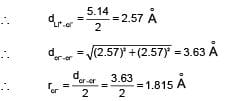Solid State & Surface Chemistry - Question 17

A solid XY has NaCI structure. If radius of X+ is 100 pm. What is the radius of Y- ion ?

Detailed Solution for Solid State & Surface Chemistry - Question 17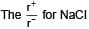= 0.414 to 0.732 (due to fcc structure)

Solid State & Surface Chemistry - Question 18

The ionic radii of Rb+ and I- are 1.46 and 2.16 Å. The most probable type of structure exhibited by it is:

Detailed Solution for Solid State & Surface Chemistry - Question 18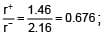Thus, it is fcc structure or NaCI type structure.

Solid State & Surface Chemistry - Question 19

Potassium has a bcc structure with nearest neighbour distance 4.52 Å . Its atomic weight is 39, its density will be :

Detailed Solution for Solid State & Surface Chemistry - Question 19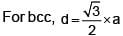or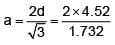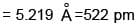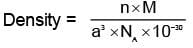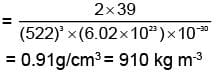Solid State & Surface Chemistry - Question 20

Which is not true in case of catalyst?

Detailed Solution for Solid State & Surface Chemistry - Question 20

Catalyst d oes not alter the equilibrium constant.

Solid State & Surface Chemistry - Question 21

Addition of FeCI3 to K4[Fe(CN)6] in dilute and cold solution gives:

Detailed Solution for Solid State & Surface Chemistry - Question 21

Fe4[Fe(CN)6]3 is Prussian blue sol of + ve charge

Solid State & Surface Chemistry - Question 22

Which is not a property of hydrophilic sols?

Detailed Solution for Solid State & Surface Chemistry - Question 22

Viscosity of hydrophilic sol is much higher than dispersion medium. Surface tension being muc h lesser than dispersion medium (water).

Solid State & Surface Chemistry - Question 23

Isoelectric point refers to the, concentration at which the colloidal particles:

Detailed Solution for Solid State & Surface Chemistry - Question 23

The sol particles at isoelectric point do not show electrophoresis.

Solid State & Surface Chemistry - Question 24

Bleeding is stopped by the application of ferric chloride. This is because:

Detailed Solution for Solid State & Surface Chemistry - Question 24

Fe3+ ion coagulates -ve sol particles of blood and seals the cut.

Solid State & Surface Chemistry - Question 25

Tanning of leather is:

Detailed Solution for Solid State & Surface Chemistry - Question 25

It is definition of tanning of leather.

Solid State & Surface Chemistry - Question 26

Which of the following is not a surfactant ?

Detailed Solution for Solid State & Surface Chemistry - Question 26

Rest all have hydrophillic head & hydrophobic tails.

Solid State & Surface Chemistry - Question 27

Which reaction gives colloidal solution?

Detailed Solution for Solid State & Surface Chemistry - Question 27

On passing H2S through a cold solution of an oxidant, colloidal sulphur is formed.

Solid State & Surface Chemistry - Question 28

How many tetrahedral holes are occupied in diamond?

Detailed Solution for Solid State & Surface Chemistry - Question 28

Diamond has ZnS structure so, 50% of tetrahedral voids are occupied with CN 4.

Solid State & Surface Chemistry - Question 29

Which of the following statements is not correct?

Detailed Solution for Solid State & Surface Chemistry - Question 29

In bcc structure CN is eight.

Solid State & Surface Chemistry - Question 30

In antifluorite structure, the negative ions:

Detailed Solution for Solid State & Surface Chemistry - Question 30

In antifluorite crystal (Na2O), the anions are arranged in cubic close packing while the cations occupy all the tetrahedral voids.

## Chemistry Mock Test Series for JEE

1 docs|26 tests
 Use Code STAYHOME200 and get INR 200 additional OFF Use Coupon Code
Information about Solid State & Surface Chemistry Page
In this test you can find the Exam questions for Solid State & Surface Chemistry solved & explained in the simplest way possible. Besides giving Questions and answers for Solid State & Surface Chemistry , EduRev gives you an ample number of Online tests for practice

1 docs|26 tests